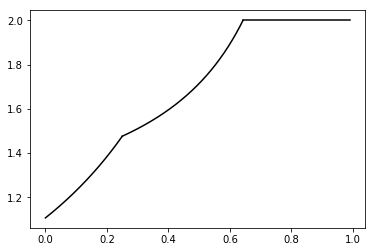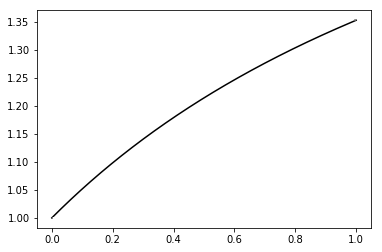# Dimension interpolation

One of the most beautiful and fundamental questions in fractal geometry is how to define the dimension of a fractal set or measure. There are many different ways to do this, each sensitive to a different geometric feature. Dimension interpolation is a new idea which seeks to understand the relationship between two given notions of dimension by viewing them as different facets of the same object: a continuum of dimensions varying from one to the other. There are three main ways to do this: the Assouad spectrum (introduced with Han Yu), intermediate dimensions (introduced with Kenneth Falconer and Tom Kempton) and the Fourier dimension spectrum (recently introduced). I wrote a survey paper on the general idea for the proceedings of Fractal Geometry and Stochastics VI, which is probably a good place to start:
► Interpolating between dimensions, arXiv
Proceedings of Fractal Geometry and Stochastics VI, Birkhäuser, Progress in Probability, 2021.
The paper in which we introduced the Assouad spectrum is here:
► New dimension spectra: finer information on scaling and homogeneity (with H. Yu), arXiv
Advances in Mathematics, 329, (2018), 273-328.
The paper in which we introduced the intermediate dimensions is here:
► Intermediate dimensions (with K. J. Falconer & T. Kempton), arXiv
Mathematische Zeitschrift, 296, (2020), 813-830.
The paper in which we introduced the Fourier dimension spectrum is here:
► The Fourier dimension spectrum and sumset type problems, arXiv
submitted.
Other work on these notions include the following:
► A new perspective on the Sullivan dictionary via Assouad type dimensions and spectra (with L. Stuart), arXiv
Bulletin of the American Mathematical Society (to appear).
► The fractal structure of elliptical polynomial spirals (with S. A. Burrell and K. J. Falconer), arXiv
Monatshefte für Mathematik, 199, (2022), 1-22.
► Projection theorems for intermediate dimensions (S. A. Burrell & K. J. Falconer), arXiv
Journal of Fractal Geometry (to appear).
► The Assouad spectrum and the quasi-Assouad dimension: a tale of two spectra (with K. E. Hare, K. G. Hare, S. Troscheit & H. Yu), arXiv
Annales Academiæ Scientiarum Fennicæ Mathematica, 44, (2019), 379-387.
► Assouad type spectra for some fractal families (with H. Yu), arXiv
Indiana University Mathematics Journal, 67, (2018), 2005-2043.Plots of the Assouad spectrum (left) and intermediate dimensions (right) for an elliptical polynomial spiral. The Assouad spectrum typically has an increasing part and then a constant part, and the phase transition between these two regimes often has particular geometric significance. The smaller phase transition typically does not appear and was a surprise observation for this family of set.

Home